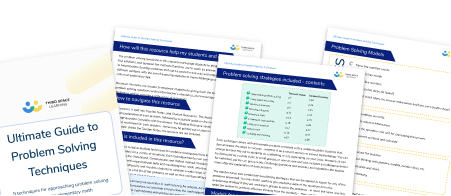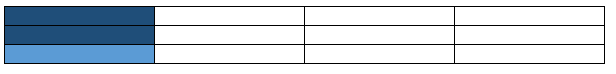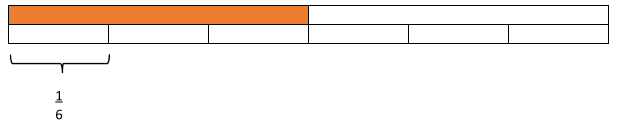Math teaching support you can trustone-on-one tutoring sessionsschools supported

[FREE] Themed Math Problems: Fall Term (Sep – Dec)

5th grade math problems and answers that link to key dates in the Fall Term, including Christmas!

# 5 Math Tricks Or Bad Habits We Should No Longer Be Teaching Elementary School Students

As teachers, we’re all looking for shortcuts and easy math tricks to teach our students but is this really the best way to help our elementary school students?

Here’s an example…

When you multiply by ten you just add a zero.

Does this make you cringe? It should as it simply isn’t true. And we know why, don’t we? If you add a zero you’re adding nothing, therefore the number doesn’t change.

Try telling that to a child who has worked it out for themselves without you ever having to utter the forbidden phrase.

As elementary teachers, we need to model good conceptual understanding in all our math lessons, rather than relying on easy math tricks that work but don’t help children to have an understanding of the ‘why’ and the ‘how’.

Here are the 5 most common bad habits I’ve identified in my role as an elementary math team leader; these are the easy math tricks teachers should no longer be using, and some ways to explain the math instead.The Ultimate Guide to Problem Solving TechniquesDownload Free Now!

### Math trick 1: using a written calculation when a mental one would do

The problem: taking the long way around

When it comes to arithmetic skills, true fluency and understanding means selecting the appropriate method of calculation.

Often, weaker mathematicians, who have learned to follow the steps of a written calculation, resort to using one wherever they see the + symbol. Even when there’s clearly a more efficient way of solving the problem.

Some teachers even encourage this behavior because ‘at least they get the right answer’, but really, this does not support their conceptual thinking further down the line.

Anyone who has graded a math assessment will have seen children arriving at an answer to 987 + 100 = using the standard algorithm, or column method. It’s basic place value and certainly something that should be done mentally, with very little thought.

Try this: children explain the method they’re using

Set an activity where all children simply have to select a method and explain why they’d use it. You can do this verbally, or in writing. Don’t even worry about solving the problems themselves. The focus here should only be on selecting a method.

For example, given a question 50 x 70, you’d hope students would not use the formal written method of multiplication. Instead, they’d use their multiplication facts and place value knowledge to get to the answer.

When you conduct this activity, make sure to include questions where children would have to use a range of methods, written and mental.

### Math trick 2: teaching written calculations without concrete representations

The problem: understanding borrowing, or regrouping

A math graduate and teacher said this to me recently: “For years, I had no idea why I crossed out a number and put a one next to the other number.”

As a child, he had not been taught why or what he was ‘borrowing’ from the next column in his subtraction written method. Nor had he been helped to understand why he had to ‘carry’ the tens when completing a written calculation for addition.

I am willing to bet good money that this lack of understanding is common.

Try this: use physical representation

The key here is to model understanding. Lower elementary school teachers often do this through manipulatives or other equipment, but we often forget to use these tools for upper elementary students.

Give children a physical representation of the exchange that takes place in an addition problem, when the tens are crossed. Or when in standard algorithm subtraction the digit being taken away is smaller than the first digit.

You can use items such as bundles of 10 straws, base ten blocks or multilink cubes in sticks of 10. Really anything that can be put in and out of groups of ten! Model the exchange that occurs in the written processes.

### Math trick 3: de-contextualising negative numbers

The problem: negative numbers seem too abstract

With many other areas of arithmetic and calculation, we tend to strip questions of any context. However, negative numbers are definitely a concept which needs to be contextualized at all times.

Children already have very little concept of negative numbers in real life (they don’t have bank balances for a start) so, as an abstract concept, it is way outside of their natural understanding.

Try this: use a vertical number line

Use a number line, a horizontal number line is OK, but a vertical one is even better. If you can then put that number line into a context the children are more likely to understand, then you’ll be onto a winner.

The best examples I’ve used are where children imagine, and eventually draw, a number line which is actually a building with floors above ground and floors below ground. The children can see, for example, that the floor below the first floor (zero) is -1 meaning one below.

This ‘number line’ is also great for setting-up and solving addition and subtraction problems: ‘If I get in the elevator at floor 5 and go down 7 floors, which floor will I arrive at?

Similar contexts such as above and below sea level will also work. The fact that thermometers too are usually encountered as a vertical number line supports this idea.

### Math trick 4: rounding numbers

The problem: rounding to 10,000 is much harder than rounding to 2 digits

Children are usually taught to round in lower elementary with a number line. We often then strip them of this representational privilege in upper elementary, making it a much more abstract process. For many, this happens before they truly understand how it all works.

Children are expected to work with larger and larger numbers and round to different amounts as the curriculum progresses.

Given this, we shouldn’t assume that because they could round to the nearest 10 in 3rd grade that they can round a 7-digit number to the nearest 10,000 in 4th grade.

Try this: go back to the number line!

Number lines to the rescue again! Use number lines throughout school, regardless of age or ability. When rounding to ten (using their place value knowledge) children will first need to find the preceding and following multiples of ten. These will be placed at either end of the number line.

Then, they should mark halfway (a multiple of 5) and then place the number they are rounding in the relevant place on the line. They will now be able to see which multiple of ten it is closest to.

It also might be worth making the number line 10 cm long so that they can accurately place the numbers. This process can be repeated for rounding to 100s, 1000s and so on.

### Math trick 5: codifying mathematical processes

The problem: statements which don’t embed conceptual understanding

“You divide by the bottom and multiply by the top!”. But why!? Sayings like this are useful because they work, but they don’t lead to a conceptual understanding of the math at work.

This is especially the case if you put these sorts of statements into knowledge organizers; the statement is internalized rather than the concept.

As teachers, we often think we have competent mathematicians on our hands because they can:

• find a fraction of an amount
• divide a fraction by another fraction – “Keep. Change. Flip” (multiply two fractions together – “Multiply the denominators together, multiply the numerators together.”
• add two fractions together – “The bottom number doesn’t change.”

We are fooling ourselves by thinking that. We’re doing the children a disservice. Without a good conceptual understanding of what happens in any one of those processes, children will struggle to apply their maxims to other problems.

Try this: use a concrete or visual representation of anything that has an easy-to-remember rule

Question: what is 3/7 of 28?

Ask children to use the squares in their book to create a grid containing 28 squares (represented below by rectangles):

They should do this using their multiplication facts knowledge. Knowing that in this example 28 divides exactly by 7, meaning that they need to draw a 4 by 7 grid (because 4 x 7 = 28). In the diagram below, each column represents one-seventh of the total (28), so children can see then that one-seventh is 4.

To find three sevenths, they could shade three of the columns and count up the total number of squares shaded. Here’s another:

Statement: 2/3 x 1/4 = _____  is the same as 2/3 of 1/4 = _____

First, children will need some background knowledge here, and the following two concepts will need to be modeled before reaching this level of question:

1. When you multiply a number by a fraction, you are actually just finding that fraction of that number.
2. When you multiply a positive number by less than a whole, the resulting answer will be smaller than the number you started with.

Ask children to draw a rectangle which is split into quarters. This is a whole. Then ask them to shade a quarter to represent the quarter in the question, like below:

Next, ask children to split the whole shape into thirds, this time with the dividing lines going horizontally. They should then shade in two-thirds of the part already shaded in, like below:A rectangle split into 12 parts, to aid students in representing the concept of two thirds

Here, some children do get confused as to which parts to shade so lots of modeling and discussion of this technique is necessary (but worthwhile).

Children should then be able to see that the whole shape has been split into 12 parts, which becomes the denominator and that two-thirds of one quarter is represented by two of those 12 parts. This can be then made into the fraction 2/12 which can be simplified to 1/6.

Obviously, this example is a 5th grade skill and a good understanding of the math behind it relies on years of quality modeling of other simpler fractions problems.

Question: 2/3 ÷ 1/4 =

For this question, children will need some squared paper to ensure accuracy. They will then need to draw a rectangle the length of the denominators multiplied. This is because to solve this we need to find a common multiple so that the two wholes are equal, accurate and comparable.

Children also need to understand that this question is asking ‘how many quarters are there in two-thirds?’

On the top, we have split the whole into thirds and below that we have split the whole into quarters, as below:

When we then partition off the two thirds (because that’s what the question is about, not a whole) we can see how many of the quarters are in (or equal to) the two thirds. In this case, there are two full quarters and then two-thirds of a quarter left.

So the answer to ‘how many quarters are there in two-thirds?’ is that there are 2 and 2/3 quarters in two-thirds.

Try this for dividing a fraction by a whole number

A similar model can obviously be used for dividing a fraction by a whole number, like this question:

Question: 1/2 ÷ 3 = _____

Here, the top bar is split into halves. Each half is then split into three. This shows that in the original whole there are six parts, so sixths. When you split a half of the whole into three parts it is a sixth of the whole. As below:A rectangle split into eight unequal parts to represent the problem ‘one half divided by three’

The complexity of the math behind problems like these might make us want to use math tricks to get students successfully answering faster. But really, these math tricks are just bad habits which support no real math understanding.

For students to truly succeed and become great mathematicians, they have to understand the concepts that underpin a problem.

Do you have students who need extra support in math?
Give your students more opportunities to consolidate learning and practice skills through personalized math tutoring with their own dedicated online math tutor.

Each student receives differentiated instruction designed to close their individual learning gaps, and scaffolded learning ensures every student learns at the right pace. Lessons are aligned with your state’s standards and assessments, plus you’ll receive regular reports every step of the way.

Personalized one-on-one math tutoring programs are available for:

The content in this article was originally written by a passionate wellbeing enthusiast and Primary Lead Practitioner Aidan Severs and has since been revised and adapted for US schools by elementary math teacher Christi Kulesza.##### Aidan Severs
Assistant Vice Principal
Aidan Severs is a passionate wellbeing enthusiast and Primary Lead Practitioner. He writes for the Third Space blog on KS2 SATs, with a focus on teacher/pupil wellbeing.
x#### The Ultimate Guide to Problem Solving Techniques